# Adventures in Kerbal Space Program, kOS & Math (Part 1)

For my “normal” blog readers, this isn’t going to make any sense. That’s why I like to think of this more as my thought dump and less as my place to placate my normal blog readers :)

So, I’ve been more or less consistently playing this game called Kerbal Space Program (KSP) since just before October 2013. It’s a game about putting little green people into space, but it’s realistic enough to keep me intrigued.

As you might know, I’m also a huge nerd, and some other huge nerd created something called Kerbal Operating System (kOS), which lets you develop programs in Kerbal Space Program to effectively control your rockets. If that sounds simple to you, you’re going to be in for a shock.

Regardless, I’m not sure what exact format this is going to take, but I wanted to record my foray into using kOS and diving into the math and science behind rockets. If you hate rockets, space or math, you’re going to absolutely despise this article.

## Executing Maneuver Nodes & Figuring Out the Rocket Equation

Let’s assume you’ve got a rocket in space. This rocket wants to change where it is in space. To do this it executes a maneuver. In KSP, this in modeled in Maneuver Nodes: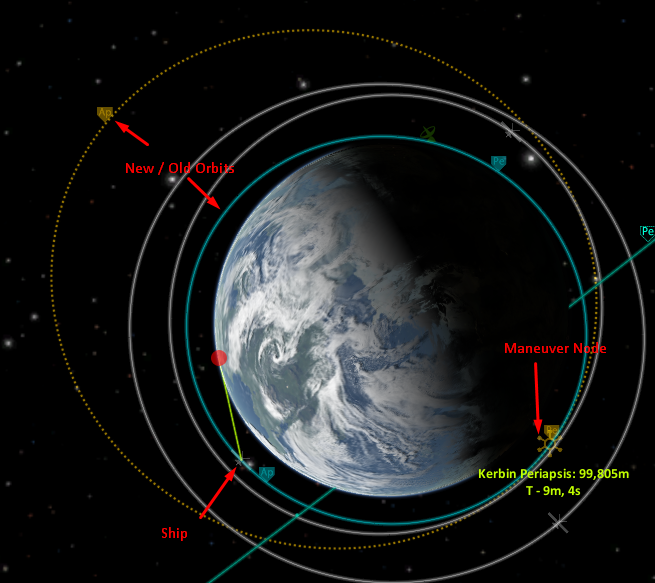I’ve got a couple of scripts written into kOS already that help me execute maneuver nodes, but they’ve given me some pretty mixed results. I think it’s because the scripts aren’t taking into account the change in acceleration due to the loss of mass of a rocket. Basically, rockets aren’t like cars. To accelerate, they shoot mass out. Hot, fiery mass.

So I’m going to do some an absurd amount of research and rewrite the script.

First off let’s break my problem down into the steps of executing a maneuver node.

1) Point at the node.
2) Start accelerating at the correct time.
3) Stop accelerating at the correct time.

That sounds pretty easy.

For #1, we’re working with nodes in kOS. Luckily there are lots of helpers for nodes, which let us write the following code:

`SET n TO NEXTNODE.`

n is now a “Node” object, which there’s lots of data in, including the vector of the node.

I did a lot of digging on vectors since I had zero experience with them, but they’re basically just directions with magnitude.

If you were to raise your hand and point at a wall in your house, you’d be identifying a direction. You could imagine that direction being drawn as a line on a graph. But vectors also represent magnitude. If you’re pointing at the wall, your finger isn’t really moving towards the wall, it’s just pointing that way. If you imagine shooting a little bolt of energy out of your finger, that bolt now has a certain velocity or magnitude in the direction you were pointing.

Good to know I guess.

kOS gives us the vector of a node fairly easily, and the cooked steering in kOS works well with vectors, so we can actually just point at the vector.

`LOCK STEERING TO n:BURNVECTOR.`

I also want to wait until I’m pointed the right way, since all the above code says is, “start turning.” I know there’s a function called VANG in kOS, which gives you the difference in angles between two vectors (you get it in a positive double, like 35.22767), and I know that one of those vectors is the burnvector. The other is given from kOS, which is SHIP:FACING:VECTOR, and means the direction your ship is facing. This is a “unit vector,” which is like a normal vector but it has a magnitude of 1. That’s more or less the written equivalent of pointing your finger at a wall.

`WAIT UNTIL VANG(SHIP:FACING:VECTOR, n:BURNVECTOR) < 2.`

So we’re basically just waiting until we point in the right direction.

Next is where I’m struggling. To figure out when to accelerate, I need to know the magnitude of the burn (AKA the dV or difference in velocity) which is simply n:burnvector:mag (the magnitude of the burnvector in m/s). But I also need to know how long it’ll take to complete the burn.

That takes a bit more doing, since rockets accelerate faster as they lose mass. Basically you’re applying the same force to a lighter object, and you get a result like hitting a baseball with a baseball bat later into the burn vs. hitting a truck early in the burn. So if I was to calculate the time it would take to move from point A to point B using my initial maximum acceleration, I’d end up at point B much sooner than anticipated. So how do I calculate this…?

Here’s my thinking.

Let’s say our initial acceleration is 10m/s^2, and our final acceleration is 20m/s^2. Since we’re expending fuel (mass) at a constant rate, I’m assuming that there’s a linear relationship between the initial acceleration and the final acceleration. So it would be reasonable to take my average acceleration into account when looking for the time it would take to get from point A to point B.

In summary, we need to know:

• Initial Max Acceleration
• Final Max Acceleration

SO! Let’s start by calculating our initial max acceleration. I had to look this up.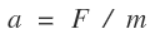Here, A is given in m/s^2 (which we want), F (Force) is expected in Newtons and m (Mass) is expected in kilograms. We can actually get these through kOS:

`SET a0 TO maxthrust / mass.`

If you check the result of maxthrust, though, you’ll find that it’s given to us in kilonewtons (1,000 Newtons), and mass is given in Tonnes (1,000 kilograms). But since they’re both 1,000 times too big and we’re dividing them, they cancel out and we’re OK.

Now we want to get our acceleration at the end of the burn, or our final max acceleration. Looking at the equation we used for the initial acceleration, the only thing that changes for our final acceleration is our mass. Unfortunately we don’t know what our final mass is, either, so we need to figure out our mass at the end of the burn.

To do this I’m using the rocket equation: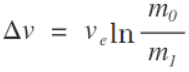So in English, the change in velocity is equal to the average exhaust speed along the axis of the engine times the natural logarithm of the difference in masses from start to finish. Exhaust speed sounds odd, but it’s just the mass expended to produce a change in velocity.

The problem is I have no idea what Ve is in this game since it’s not listed anywhere, and it’s clearly specific to each engine. After some digging I found that Ve is equal to the Isp times the force of gravity on earth (Ve = Isp * g0). Isp is the value listed on an engine in KSP as Specific Impulse: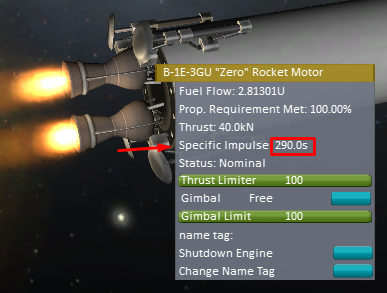And I know both Isp and g0 (g zero), so I can solve for Ve.

On a sidenote, holy shit is that Ve and Isp thing confusing. Right now I know of three useful conceptions of g in the sense of gravity. Big G, for the gravitational constant, little g for F = (G * m1 * m2) / r^2, and now another little g that represents the force of gravity on earth. Are these last two actually not different? E.g., does Isp change as little g changes? That doesn’t make sense to me since it represents the mass ejected from the rocket in this case, and that doesn’t seem like it should change based on how far you are from a planet, so only Earth standard gravity seems to make sense.

ANYWAY. I’m still digging myself deeper, because while I was initially just trying to get my final acceleration, I wound up needing my final mass, and now I have to get both the effective Isp and Earth (Kerbin) standard gravity just to solve Ve… which is what we’re doing now.

So say I have 2 active engines on my ship, one has an Isp of 120 and a thrust of 20kN, the other has an Isp of 300 and a thrust of 40kN. The effective Isp is closer to 300 than 120 because the engine with the higher Isp is using twice as much fuel as the engine with the lower Isp. This is more or less a weighted average, so I figure that: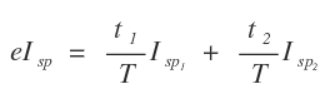Where t is the thrust of the engine and T is the total thrust of all engines. Just keep adding engines in this way. Codewise, keeping in mind that deactivated engines have an Isp of 0:

```SET eIsp TO 0.
LIST engines IN my_engines.
FOR eng IN my_engines {
IF eng:isp > 0 {
SET eIsp TO eIsp + eng:maxthrust / maxthrust * eng:isp.
}
}
```

Thinking about it, since eng:isp for a deactivated engine is going to be 0 anyway, a deactivated engine wouldn’t add anything to the eIsp because anything times 0 is 0, so we can get rid of the conditional.

```SET eIsp TO 0.
LIST engines IN my_engines.
FOR eng IN my_engines {
SET eIsp TO eIsp + eng:maxthrust / maxthrust * eng:isp.
}```

For Kerbin’s standard gravity, I’m pretty sure we can just use 9.81m/s^2. Am I wrong here?**

OK, I’m NOT wrong in real life, but apparently in KSP they use 9.82 m/s^2, so I’m updating this post to match.

So:

`SET Ve TO eIsp * 9.82.`

Sweet christ. Alright, so I’m back to solving the rocket equation, but it’s out of order. I’ve finally got everything I need in this equation except for the final mass:I have no idea how to rearrange this to solve for m1, mostly because I’m pretty clueless about ln (natural logarithm or log to base e, where e is some weird constant). Luckily Wikipedia gives this: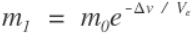Which, let’s be honest, looks pretty intense anyway. Since I have all of these values though it’s just a matter of throwing them in, remembering that e is Euler’s number, which kOS gives us as a constant. What is Euler’s number? I don’t know, but I’ve seen the movie and he’s not in class.

`SET final_mass TO mass*CONSTANT():e^(-1*n:BURNVECTOR:MAG/Ve).`

How ’bout them apples.

OK, now I’ve got no idea where I’m at. We were going to do this:

1) Point at the node.
2) Start accelerating at the correct time.
3) Stop accelerating at the correct time.

Our code right now is:

```// Point at the node.
SET n TO NEXTNODE.
LOCK STEERING TO n:BURNVECTOR.
WAIT UNTIL VANG(SHIP:FACING:VECTOR, n:BURNVECTOR) < 2.

// Get initial acceleration.
SET a0 TO maxthrust / mass.

// In the pursuit of a1...
// What's our effective ISP?
SET eIsp TO 0.
LIST engines IN my_engines.
FOR eng IN my_engines {
SET eIsp TO eIsp + eng:maxthrust / maxthrust * eng:isp.
}

// What's our effective exhaust velocity?
SET Ve TO eIsp * 9.82.

// What's our final mass?
SET final_mass TO mass*CONSTANT():e^(-1*n:BURNVECTOR:MAG/Ve).```

OK, kind of clear again. So to get a1, we just do the acceleration equation again, but this time we use the final mass instead of initial mass:

`SET a1 TO maxthrust / final_mass.`

And finally all I wanted from all of this was how long it’d take to burn through my node. Apparently this comes from a SUVAT equation, or an equation of motion, of which we’re using this guy: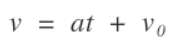Which means that the final velocity is equal to the acceleration times the amount of time you’re accelerating plus the initial velocity. We’re trying to solve for t here, so if my steadily improving English major algebra doesn’t fail me: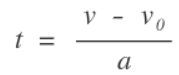AKA: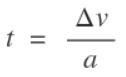So we decided earlier that the average acceleration can count as my a value in there, which is just (a0 + a1) / 2, and the deltaV has always been n:BURNVECTOR:MAG, which gives us:

`SET t TO n:BURNVECTOR:MAG / ((a0 + a1) / 2).`

That’s the amount of time it’ll take to burn through a maneuver node with a rocket that (by definition) changes mass as it accelerates and has varying degrees of efficiency in any number of engines.

Now say the maneuver happens at time A. We want to start out burn before that time and end it afterwards, such that we’re halfway through our burn by exactly time A. The start time of our burn, then, is A – t/2, and the end time of our burn is exactly A + t/2. We can get the exact time of the node by asking kOS for n:ETA, and we need to make sure that we’re doing this relative to some actual timeframe (TIME:SECONDS). So we’ve got:

```SET start_time TO TIME:SECONDS + n:ETA - t/2.
SET end_time TO TIME:SECONDS + n:ETA + t/2.```

To actually complete the burn, we just say:

```WAIT UNTIL TIME:SECONDS >= start_time.
LOCK throttle TO 1.
WAIT UNTIL TIME:SECONDS >= end_time.
LOCK throttle TO 0.```

Since that “WAIT UNTIL” looks makes its check periodically, there’s some room for error on the start/end times, but it’s not much.

Finally, and I’m not 100% sure about this, but since all of this math assumes that you can change your velocity from v0 to v1 instantly, and in our case we’re doing it over the time of the burn, we’re probably losing some efficiency and there should be a tiny bit of dV left over for us to burn through, especially on longer burns. I’ve got some customizations to write for this (next time) anyway, so I’ll leave solving that issue for later.

Our final code is:

```// Point at the node.
SET n TO NEXTNODE.
LOCK STEERING TO n:BURNVECTOR.
WAIT UNTIL VANG(SHIP:FACING:VECTOR, n:BURNVECTOR) < 2.

// Get initial acceleration.
SET a0 TO maxthrust / mass.

// In the pursuit of a1...
// What's our effective ISP?
SET eIsp TO 0.
LIST engines IN my_engines.
FOR eng IN my_engines {
SET eIsp TO eIsp + eng:maxthrust / maxthrust * eng:isp.
}

// What's our effective exhaust velocity?
SET Ve TO eIsp * 9.82.

// What's our final mass?
SET final_mass TO mass*CONSTANT():e^(-1*n:BURNVECTOR:MAG/Ve).

// Get our final acceleration.
SET a1 TO maxthrust / final_mass.
// All of that ^ just to get a1..

// Get the time it takes to complete the burn.
SET t TO n:BURNVECTOR:MAG / ((a0 + a1) / 2).

// Set the start and end times.
SET start_time TO TIME:SECONDS + n:ETA - t/2.
SET end_time TO TIME:SECONDS + n:ETA + t/2.

// Complete the burn.
WAIT UNTIL TIME:SECONDS >= start_time.
LOCK throttle TO 1.
WAIT UNTIL TIME:SECONDS >= end_time.
LOCK throttle TO 0.```

### 4 thoughts on “Adventures in Kerbal Space Program, kOS & Math (Part 1)”

1.zilti

“apparently in KSP they use 9.82 m/s^2” Hmm, so they use a different gravitational constant? Because, according to Wikipedia, the constant is 6.67408 * 10^(-11). Calculating G * SHIP:BODY:MASS / SHIP:BODY:RADIUS ^ 2 then gives me 9.81. Weird. By the way, thanks a ton for the post, it saved me a lot of time searching around :)

1.Yeah that one I’m not sure about. I remember asking the kOS community about it and that’s what they told me. The burns you get out of it are super accurate though, not accounting for cosine losses. But yeah no worries! I feel like I’ll never use this again for the rest of my life, but it was fun to figure out :)

2.zilti

…by the way, there’s one thing you’ve “missed”. Of course when it’s not the last stage you’re on, in “FOR eng IN my_engines” you should also ensure that the engines you’re adding are all in the active stage (“IF eng:stage = stage:number”).

1.Haha thanks :) I actually wrote about why I skipped it; eng:isp is 0 for deactivated engines, so it cuts itself out. I should probably have annotated that in the code for clarity, but I was using the article for my annotation :D